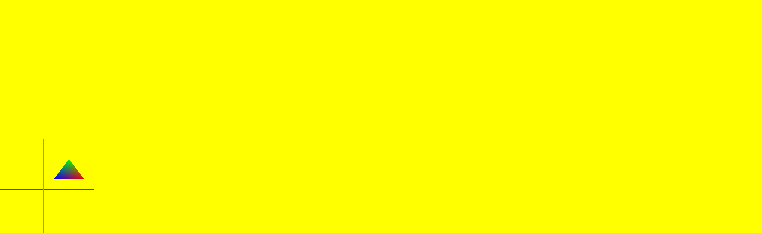# Incorrect Position of viewport in OpenGL + Qt

• Hello,
I am working on a CAD type application,where i am using multiple QOpenglWidgets. I am trying to render a SAMPLE Triangle but the viewport position is incorrect.

//This the resize function-------------------------------------------------

void resizeEvent(QResizeEvent * event)
{
unsigned int width=event->size().width();
unsigned int height=event->size().height();

if (height == 0) height = 1;
GLfloat aspect = (GLfloat)width / (GLfloat)height;
glViewport(0, 0, width, height);
glMatrixMode(GL_PROJECTION);

if (width >= height)
{
// aspect >= 1, set the height from -1 to 1, with larger width
gluOrtho2D(-1.0 * aspect, 1.0 * aspect, -1.0, 1.0);
}
else
{
// aspect < 1, set the width to -1 to 1, with larger height
gluOrtho2D(-1.0, 1.0, -1.0 / aspect, 1.0 / aspect);
}
glMatrixMode(GL_MODELVIEW);
}

// And from PaintEvent i am calling this-------------------

void SAMPLE_OPENGL(float Ty)
{

glClearColor(1,1,0,1);
glClear(GL_COLOR_BUFFER_BIT | GL_DEPTH_BUFFER_BIT);

qDebug("~TRIANGLE~~~~");

glMatrixMode(GL_MODELVIEW); // To operate on Model-View matrix
glLoadIdentity(); // Reset the model-view matrix

glBegin(GL_LINES); // sample X,Y axis (in immediate mode) (Just to check)
glColor3f(1,0,0); //X
glVertex2f(-1.0f, 0.0f);
glVertex2f( 3.0f, 0.0f);
glColor3f(0,1,0); //Y
glVertex2f( 0.0f, 1.0f);
glVertex2f( 0.0f, -1.0f);
glEnd();

glTranslatef(0, Ty, 0.0f); // Translate left and up
glBegin(GL_TRIANGLES); // Each set of 3 vertices form a triangle
glColor3f(0.0f, 0.0f, 1.0f); // Blue
glVertex2f(0.2f, 0.2f);
glColor3f(1.0f, 0.0f, 0.0f); // Red
glVertex2f(0.8f, 0.2f);
glColor3f(0.0f, 1.0f, 0.0f); // Green
glVertex2f(0.5f, 0.6f);
glEnd();
glPopMatrix();

}

//Note there are multiple QOpenglWidgets
//Here is the screenshot of output• Hi and welcome to devnet,

From the looks of it you are using both glu and the fixed pipeline.

Since you are starting a new project, may a suggest taking a look at the Qt OpenGL examples and their use of current technologies like shaders ?